# OIympiad Test: Mathematics - 4

Test Description

## 10 Questions MCQ Test Mathematics Olympiad for Class 1 | OIympiad Test: Mathematics - 4

OIympiad Test: Mathematics - 4 for Class 1 2023 is part of Mathematics Olympiad for Class 1 preparation. The OIympiad Test: Mathematics - 4 questions and answers have been prepared according to the Class 1 exam syllabus.The OIympiad Test: Mathematics - 4 MCQs are made for Class 1 2023 Exam. Find important definitions, questions, notes, meanings, examples, exercises, MCQs and online tests for OIympiad Test: Mathematics - 4 below.
 1 Crore+ students have signed up on EduRev. Have you?
OIympiad Test: Mathematics - 4 - Question 1

### Varun made 25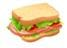sandwiches and distributed them equally among his 5 friends. How many sandwiches did each of his friends get?

Detailed Solution for OIympiad Test: Mathematics - 4 - Question 1

25 ÷ 5 = 5
Therefore, 5 sandwiches did each of his friends get.

OIympiad Test: Mathematics - 4 - Question 2

### Mona’s father has given her 100 as a birthday gift. Which of the following toys can she buy?

Detailed Solution for OIympiad Test: Mathematics - 4 - Question 2

OIympiad Test: Mathematics - 4 - Question 3

### Pooja bought the given tennis ball. She gave ₹ 50 to the shopkeeper. how much amount of money did she get back?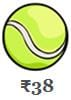Detailed Solution for OIympiad Test: Mathematics - 4 - Question 3

Total amount she has ₹ 50
She gave = ₹ 38
She get back = 50 - 38 = ₹ 12

OIympiad Test: Mathematics - 4 - Question 4

Shreya bought 12 cupcakes on monday, 10 cupcakes on tuesday and 34 cupcakes on wednesday. How many cupcakes did she buy altogether?

Detailed Solution for OIympiad Test: Mathematics - 4 - Question 4

12 + 10 + 34 = 56

OIympiad Test: Mathematics - 4 - Question 5

Riya had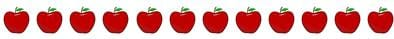She ate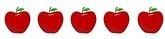________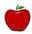are left with her.

Detailed Solution for OIympiad Test: Mathematics - 4 - Question 5

12 - 5 = 7
Therefore, 7 apples are left with her.

OIympiad Test: Mathematics - 4 - Question 6

A monster has 5 eyes. 5 such monsters will have _________ eyes.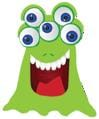Detailed Solution for OIympiad Test: Mathematics - 4 - Question 6

5 x 5 = 25
Therefore, 5 such monsters will have 25 eyes.

OIympiad Test: Mathematics - 4 - Question 7

Prateek bought a cold drink can as shown in the picture. The shape of the can is _________.Detailed Solution for OIympiad Test: Mathematics - 4 - Question 7

The shape of the can is Cylinder.

OIympiad Test: Mathematics - 4 - Question 8

The weight of Priya'sis equal to the weight of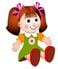The weight ofis equal to the weight of _________..

Detailed Solution for OIympiad Test: Mathematics - 4 - Question 8

The weight ofis equal to the weight of 8.

OIympiad Test: Mathematics - 4 - Question 9

Vinay had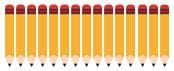He used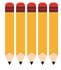Which of  the following number sentences represents the number of pencils left with him?

Detailed Solution for OIympiad Test: Mathematics - 4 - Question 9

Total Number of pencils = 15
He used = 5
Therefore, 15 - 5 = 10 pencils left with him.

OIympiad Test: Mathematics - 4 - Question 10

_______ is taller than ali but shorter than ella.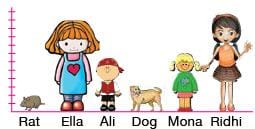Detailed Solution for OIympiad Test: Mathematics - 4 - Question 10

Mona is taller than ali but shorter than ella.

## Mathematics Olympiad for Class 1

8 videos|19 docs|15 tests
 Use Code STAYHOME200 and get INR 200 additional OFF Use Coupon Code